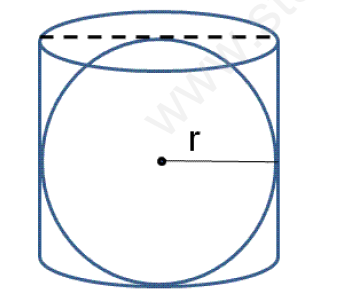# CBSE Class 9 Mathematics Surface areas and Volumes Assignment Set C

Read and download free pdf of CBSE Class 9 Mathematics Surface areas and Volumes Assignment Set C. Get printable school Assignments for Class 9 Surface areas and Volumes. Standard 9 students should practise questions and answers given here for Surface areas and Volumes in Grade 9 which will help them to strengthen their understanding of all important topics. Students should also download free pdf of Printable Worksheets for Class 9 Surface areas and Volumes prepared as per the latest books and syllabus issued by NCERT, CBSE, KVS and do problems daily to score better marks in tests and examinations

## Surface areas and Volumes Assignment for Class 9

Class 9 Surface areas and Volumes students should refer to the following printable assignment in Pdf in standard 9. This test paper with questions and answers for Grade 9 Surface areas and Volumes will be very useful for exams and help you to score good marks

### Class 9 Surface areas and Volumes Assignment Pdf

Surface Areas and Volumes

Q 1 Find the area enclosed between two concentric circles of radii 4 cm and 3 cm.

Q 2 The diameter of a garden roller is 1.4 m and it is 2 m long. How much area will it cover in 5 revolutions?

Q 3 A cuboid has total surface area of 40 sq m and its lateral surface area is 26 sq m. Find the area of base.

Q 4 Three metal cubes whose edges measure 3 cm, 4 cm and 5 cm respectively are melted to form a single cube. Find the edge of the new cube. Also find the surface area of the new cube.

Q 5 An iron pipe 20 cm long has exterior diameter equal to 50 cm. If the thickness of the pipe is 1cm, find the whole surface area of the pipe.

Q 6 The lateral surface of a cylinder is equal to the curved surface of a cone. If the radius is the same, find the ratio of the height of the
cylinder and slant height of the cone.

Q 7 A right circular cylinder just enclosed a sphere of radius r as shown in figure find the surface area of the sphere , curved surface area of the cylinder and also their ratio.Q 8 A godown is in the form of a cuboid measuring 60 m x 40 m x 20 m. How many cuboidal boxes can be stored in it if the volume of one box 0.8 m3?

Click on link below to download CBSE Class 9 Mathematics Surface areas and Volumes Assignment Set C

 CBSE Class 9 Mathematics Surface areas and Volumes Assignment Set A CBSE Class 9 Mathematics Surface areas and Volumes Assignment Set B CBSE Class 9 Mathematics Surface areas and Volumes Assignment Set C CBSE Class 9 Mathematics Surface areas and Volumes Assignment Set D